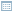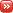Posted by Konstantin 25.02.2013

If anyone tells you he or she understands probability theory, do not believe them. That person simply does not know enough of it to admit, that probability theory is riddled with paradoxes, where common sense must step aside and wait in silence, or your brain will hurt. Substring statistics is probably among the lesser-known, yet magically unintuitive examples.

Consider a sequence of random coin flips. Each coin flip is either a "heads" or a "tails", hence the sequence might written down as a sequence of `H` and `T`-s: `HTHTHHTT...`

It is easy to show that the probability of the sequence to begin with, say, `HHH` is equal to P(`HHH`) = 1/8th, as is the case with any other three-letter combination: P(`HHT`) = P(`THH`) = P(`THT`) = 1/8, etc. Moreover, by symmetry, the probability of seeing a particular three-letter combination at any fixed position in the sequence is still always 1/8-th. All three-letter substrings seem to be equivalent here.

But let us now play a game, where we throw a coin until we see a particular three-letter combination. To be more specific, let us wait until either `HHT` or `HHH` comes up. Suppose I win in the first case and you win in the second one. Obviously, the game first proceeds until two heads are flipped. Then, whichever coin flip comes up next determines the winner. Sounds pretty fair, doesn't it? Well, it turns out that, surprisingly, if you count carefully the expected number of coin flips to obtain `HHT`, it happens to be 8, whereas for `HHH` it is 14! Ha! Does it mean I have an advantage? Suprisingly again, no. The probability of `HHT` occuring before `HHH` in any given sequence is still precisely 0.5 and, as we reasoned initially, the game is still fair.

We can, however, construct even more curious situations with four-letter combinations. Suppose I bet on `HTHT` and you bet on `THTT`.  The expected number of coin flips to obtain my combination can be computed to be 20. The expected number of flips to get your combination is smaller: 18 flips. However, it is still more probable (64%) that my combination will happen before yours!

If this is not amusing enough, suppose that four of us are playing such a game. Player A bets on the string `THH`, Player B bets on `HHT`, player C on `HTT` and player D on `TTH`. It turns out that A's combination will occur earlier than B's with probability 75%. B's combination, however, wins over C's with probability 66.7%. C's combination, though, wins over D's with probability 75%. And, to close the loop, D wins over A with probability 66.7%! This is just like the nontransitive dice.

Hopefully, you are amazed enough at this point to require an explanation for how this all might happen. Let me leave it to you as a small puzzle:

• Explain in simple terms, how can it happen so that the expected time to first occurrence of otherwise equiprobable substrings may be different?
• Explain in simple terms, how can it be so that one substring has higher than 50% chance of occuring earlier than some other substring.
• Finally, explain why the two effects above are not strictly related to each other.

PS: The theory used to compute actual probabilities and expected times to occurrence of a substring is elegant yet somewhat involved. For the practically-minded, here is the code to check the calculations.

Posted by Konstantin @ 12:43 am

Please note: Comment moderation is enabled and may delay your comment. There is no need to resubmit your comment.

### Calendar

February 2013
M T W T F S S
« Jan   Apr »
123
45678910
11121314151617
18192021222324
25262728